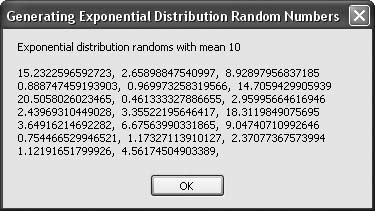# Recipe 6.30. Generating Exponential-Distribution Random Numbers

#### Problem

You need to generate a sequence of pseudorandom numbers with an exponential distribution given the distribution's mean.

#### Solution

Sample code folder: Chapter 06\RepeatRandom

The BetterRandom class (see Recipe 6.26) sports a GetNextExp() function. One parameter passed to this function defines the mean of the exponentially distributed return values:

` GetNextExp(mean) `

#### Discussion

The following code creates a new instance of the BetterRandom object, which it then uses to generate 20 pseudorandom double-precision numbers with the desired exponential distribution. As a programming exercise you might consider changing this code to display the mean of the returned values, to compare the results with the goal.

The generator object is created without passing a string to initialize the generator, so a unique sequence is created every time this program is run:

` Dim result As New System.Text.StringBuilder Dim generator As New BetterRandom Dim mean As Double = 10 Dim counter As Integer result.Append("Exponential distribution randoms with mean ") result.AppendLine(mean) result.AppendLine() For counter = 1 To 20    ' ----- Add one random number.    result.Append(generator.GetNextExp(mean))    If ((counter Mod 3) = 0) Then       ' ----- Group on distinct lines periodically.       result.AppendLine()    Else       result.Append(", ")    End If Next counter MsgBox(result.ToString()) `

Figure 6-30 shows the results of generating the 20 pseudorandom double-precision exponential-distribution numbers.

##### Figure 6-30. Pseudorandom exponentially distributed numbers generated by the BetterRandom objectRecipe 6.26 shows the full code for the BetterRandom class.

There are many good references on the Web to learn more about random number generation (see, for example, http://random.mat.sbg.ac.at).Visual Basic 2005 Cookbook: Solutions for VB 2005 Programmers (Cookbooks (OReilly))
ISBN: 0596101775
EAN: 2147483647
Year: 2006
Pages: 400

Similar book on Amazon